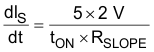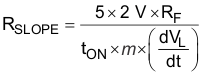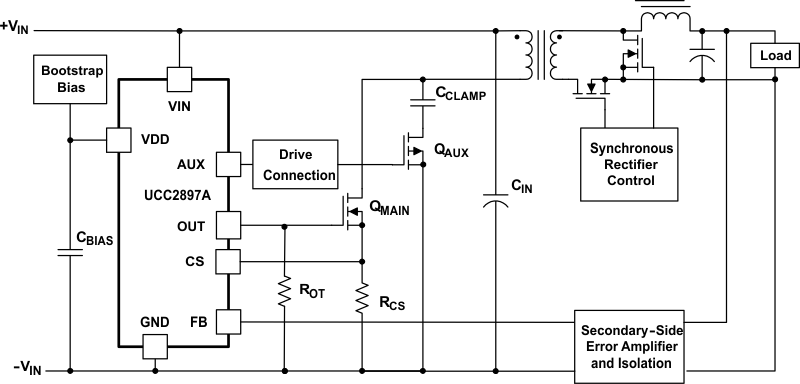SLUS829G August   2008  – February 2020

PRODUCTION DATA.

1. Features
2. Applications
3. Description
1.     Device Images
4. Revision History
5. Device Options
6. Pin Configuration and Functions
7. Specifications
8. Detailed Description
1. 8.1 Overview
2. 8.2 Functional Block Diagram
3. 8.3 Feature Description
1. 8.3.1 Detailed Pin Descriptions
2. 8.3.2 JFET Control and UVLO
3. 8.3.3 Line Undervoltage Protection
4. 8.3.4 Line Overvoltage Protection
5. 8.3.5 Pulse Skipping
6. 8.3.6 Synchronization
7. 8.3.7 Gate Drive Connection
8. 8.3.8 Bootstrap Biasing
4. 8.4 Device Functional Modes
9. Application and Implementation
1. 9.1 Application Information
2. 9.2 Typical Application
1. 9.2.1 Design Requirements
2. 9.2.2 Detailed Design Procedure
3. 9.2.3 Application Curves
10. 10Power Supply Recommendations
11. 11Layout
12. 12Device and Documentation Support
13. 13Mechanical, Packaging, and Orderable Information

• RGP|20
• PW|20
• RGP|20
• PW|20

#### 9.2.2.6 Current Sense and Slope Compensation

The UCC2897A offers onboard user-programmable slope-compensation. The programming of the correct amount of slope compensation is accomplished by the appropriate selection of two external resistors, RF and RSLOPE.

First, the current-sense filter-resistor value (RF) must be calculated based on the desired filtering of the current-sense signal. The filter consists of two components, CF and RF. The CF filter capacitor is connected between the CS pin and the GND pin. While the value of CF is selected freely as the first step of the filter design, the value of CF is minimized to avoid filtering the slope compensation current exiting the CS pin. The recommended range for the filter capacitance is between 50 pF and 270 pF. The value of the filter resistor is calculated from the filter capacitance and the desired filter corner frequency fF.

Equation 26.After RF is defined, RSLOPE is calculated. The amount of slope compensation is defined by the stability requirements of the inner-peak current-loop of the control algorithm and is measured by the number m. When the slope of the applied compensation ramp equals the down-slope of the output-inductor current waveform reflected across the primary-side current-sense resistor (dVL / dt), m = 1. The minimum value of m is 0.5 to prevent current-loop instability. The best current-mode performance is achieved around m = 1. The increase of m moves the control closer to the voltage-mode control operation.

In the UCC2897A controllers, slope compensation is implemented by sourcing a linearly-increasing current at the CS pin. When this current passes through the current-sense filter resistor (RF), the current converts to a slope-compensation ramp which is characterized by (dVS / dt). The (dVS / dt) of the slope-compensation current is defined by RSLOPE according to Equation 27.

Equation 27.where

• 2 V is the peak-to-peak ramp amplitude of the internal oscillator waveform
• 5 is the multiplication factor of the internal current mirror

The voltage equivalent of the compensation ramp (dVS / dt) is obtained easily by multiplying with RF. After introducing the application specific m and (dVL / dt) values, Equation 28 is rearranged for RSLOPE.

Equation 28.Figure 29. Active Clamp Forward Converter